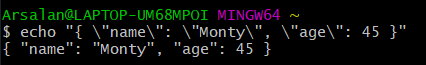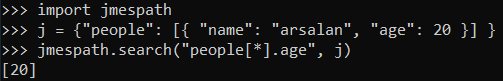# Working With JSON Data in Python

• Difficulty Level : Easy
• Last Updated : 03 Jun, 2022

JSON is JavaScript Object Notation. It means that a script (executable) file which is made of text in a programming language, is used to store and transfer the data. Python supports JSON through a built-in package called JSON. To use this feature, we import the JSON package in Python script. The text in JSON is done through quoted-string which contains the value in key-value mapping within { }. It is similar to the dictionary in Python. JSON shows an API similar to users of Standard Library marshal and pickle modules and Python natively supports JSON features. For example:

## Python3

 `# Python program showing ` `# use of json package`   `import` `json`   `# {key:value mapping}` `a ``=``{``"name"``:``"John"``,` `   ``"age"``:``31``,` `    ``"Salary"``:``25000``}`   `# conversion to JSON done by dumps() function` ` ``b ``=` `json.dumps(a)`   `# printing the output` `print``(b)`

Output:

`{"age": 31, "Salary": 25000, "name": "John"}`

As you can see, JSON supports primitive types, like strings and numbers, as well as nested lists, tuples, and objects

## Python3

 `# Python program showing that` `# json support different primitive` `# types`   `import` `json`   `# list conversion to Array` `print``(json.dumps([``'Welcome'``, ``"to"``, ``"GeeksforGeeks"``]))`   `# tuple conversion to Array` `print``(json.dumps((``"Welcome"``, ``"to"``, ``"GeeksforGeeks"``)))`   `# string conversion to String` `print``(json.dumps(``"Hi"``))`   `# int conversion to Number` `print``(json.dumps(``123``))`   `# float conversion to Number` `print``(json.dumps(``23.572``))`   `# Boolean conversion to their respective values` `print``(json.dumps(``True``))` `print``(json.dumps(``False``))`   `# None value to null` `print``(json.dumps(``None``))`

Output:

```["Welcome", "to", "GeeksforGeeks"]
["Welcome", "to", "GeeksforGeeks"]
"Hi"
123
23.572
true
false
null```

### Serializing JSON:

The process of encoding JSON is usually called serialization. This term refers to the transformation of data into a series of bytes (hence serial) to be stored or transmitted across a network. To handle the data flow in a file, the JSON library in Python uses dump() function to convert the Python objects into their respective JSON object, so it makes it easy to write data to files. See the following table given below.

### Example: Serialization

Consider the given example of a Python object.

## Python3

 `var ``=` `{ ` `      ``"Subjects"``: {` `                  ``"Maths"``:``85``,` `                  ``"Physics"``:``90` `                   ``}` `      ``}`

Using Python’s context manager, create a file named Sample.json and open it with write mode.

## Python3

 `with ``open``(``"Sample.json"``, ``"w"``) as p:` `     ``json.dump(var, p)`

Here, the dump() takes two arguments first, the data object to be serialized, and second the object to which it will be written(Byte format).

### Deserializing JSON:

Deserialization is the opposite of Serialization, i.e. conversion of JSON objects into their respective Python objects. The load() method is used for it. If you have used JSON data from another program or obtained it as a string format of JSON, then it can easily be deserialized with load(), which is usually used to load from a string, otherwise, the root object is in a list or dict.

## Python3

 `with ``open``(``"Sample.json"``, ``"r"``) as read_it:` `     ``data ``=` `json.load(read_it)`

## Python3

 `json_var ``=``"""` `{` `    ``"Country": {` `        ``"name": "INDIA",` `        ``"Languages_spoken": [` `            ``{` `                ``"names": ["Hindi", "English", "Bengali", "Telugu"]` `            ``}` `        ``]` `    ``}` `}` `"""` `var ``=` `json.loads(json_var)`

### Encoding and Decoding:

Encoding is defined as converting the text or values into an encrypted form that can only be used by the desired user through decoding it. Here encoding and decoding is done for JSON (object)format. Encoding is also known as Serialization and Decoding is known as Deserialization. Python has a popular package for this operation. This package is known as Demjson. To install it follow the steps below.

For Windows:

`pip install demjson`

For Ubuntu:

``` sudo apt-get update
sudo apt-get install python-demjson```

Encoding: The encode() function is used to convert the python object into a JSON string representation.

Syntax:

`demjson.encode(self, obj, nest_level=0) `

Example 1: Encoding using demjson package.

## Python3

 `# storing marks of 3 subjects` `var ``=` `[{``"Math"``: ``50``, ``"physics"``:``60``, ``"Chemistry"``:``70``}]` `print``(demjson.encode(var))`

Output:

`[{"Chemistry":70, "Math":50, "physics":60}]`

Decoding: The decode() function is used to convert the JSON object into python-format type.

Syntax:

`demjson.decode(self, obj)`

Example 2: Decoding using demjson package

## Python3

 `var ``=` `'{"a":0, "b":1, "c":2, "d":3, "e":4}'` `text ``=` `demjson.decode(var)`

Output:

`{'a': 0, 'b': 1, 'c': 2, 'd': 3, 'e': 4}`

Example 3: Encoding using iterencode package

## Python3

 `# Other Method of Encoding` `json.JSONEncoder().encode({``"foo"``: [``"bar"``]})` `'{"foo": ["bar"]}'`   `# Using iterencode(object) to encode a given object.` `for` `i ``in` `json.JSONEncoder().iterencode(bigobject):` `    ``mysocket.write(i)`

Example 4: Encoding and Decoding using dumps() and loads().

## Python3

 `# To encode and decode operations` `import` `json` `var ``=` `{``'age'``:``31``, ``'height'``:``6``}` `x ``=` `json.dumps(var)` `y ``=` `json.loads(x)` `print``(x)` `print``(y)`   `# when performing from a file in disk` `with ``open``(``"any_file.json"``, ``"r"``) as readit:` `    ``x ``=` `json.load(readit)` `print``(x)`

### Command-Line Usage

The JSON library can also be used from the command-line, to validate and pretty-print your JSON.

`\$ echo "{ \"name\": \"Monty\", \"age\": 45 }"`### Searching through JSON with JMESPath

JMESPath is a query language for JSON. It allows you to easily obtain the data you need from a JSON document. If you ever worked with JSON before, you probably know that it’s easy to get a nested value. For example, doc[“person”][“age”] will get you the nested value for age in a document.

First, install jmespath :

`\$ pip3 install jmespath`### Real-World Example:

Let us take a real-life example of the implementation of the JSON in python. A good source for practice purposes is JSON_placeholder, it provides a great API requests package which we will be using in our example. To get started, follow these simple steps. Open Python IDE or CLI and create a new script file, name it sample.py.

## Python3

 `import` `requests` `import` `json`   `# Now we have to request our JSON data through` `# the API package` `res ``=` `requests.get(``"https://jsonplaceholder.typicode.com / todos"``)` `var ``=` `json.loads(res.text)`   `# To view your Json data, type var and hit enter` `var`   `# Now our Goal is to find the User who have ` `# maximum completed their task !!` `# i.e we would count the True value of a ` `# User in completed key.` `# {` `    ``# "userId": 1,` `    ``# "id": 1,` `    ``# "title": "Hey",` `    ``# "completed": false,  # we will count` `                           ``# this for a user.` `# }`   `# Note that there are multiple users with ` `# unique id, and their task have respective` `# Boolean Values.`   `def` `find(todo):` `    ``check ``=` `todo[``"completed"``]` `    ``max_var ``=` `todo[``"userId"``] ``in` `users` `    ``return` `check ``and` `max_var`   `# To find the values.`   `Value ``=` `list``(``filter``(find, todos))`   `# To write these value to your disk`   `with ``open``(``"sample.json"``, ``"w"``) as data:` `    ``Value ``=` `list``(``filter``(keep, todos))` `    ``json.dump(Value, data, indent ``=` `2``)`